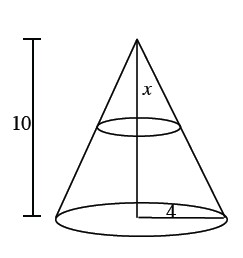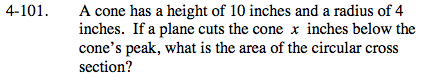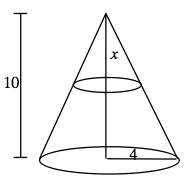### Home > PC > Chapter 4 > Lesson 4.2.3 > Problem4-101

4-101.

A cone has a height of 10 inches and a radius of 4 inches. If a plane cuts the cone x inches below the cone's peak, what is the area of the circular cross section? Homework Help ✎Find r first in terms of x using proportions.

$\frac{10}{4}=\frac{x}{r}\text{ Solve for } r\text{ in terms of }x.$Area = π r2
Substitute your result for r into the area formula.